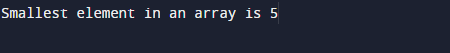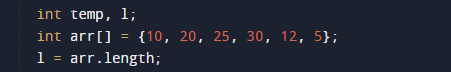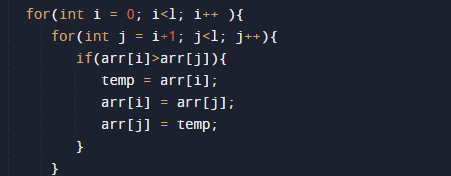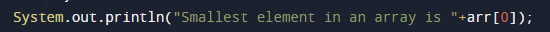# Java to Program Find Smallest Number in an array

In this tutorial you will learn about the Java to Program Find Smallest Number in an array and its application with practical example.

In this tutorial, we will learn to create a Java to Program Find 2nd Smallest Number in an array using java programming.

## Prerequisites

Before starting with this tutorial, we assume that you are best aware of the following Java programming concepts:

• Java Operators.
• Basic Input and Output function in Java.
• Class and Object in Java.
• Basic Java programming.
• If-else statements in Java.
• For loop in Java.

## Java to Program Find  Smallest Number in an array

In this program we will find smallest element in an array using nested for loop. We would first declared and initialized the required variables. Next, we will find smallest number in an array. Lets have a look at the code.

## Output

Smallest in an arrayIn the above program, we have first declared and initialized a set variables required in the program.

• arr[]= it will hold array values
• l=  it will hold length of an array.
• i and j for iteration
• temp = it will hold temporary value of an array.

After declaring variables we initiate values in  an array[]with the help of length function we  will find the length of an array .

after that with the help or nested for loop we will find smallest element in array as shown in image below## Smallest in an array

• Here first we assign first value of an array to variable temp.
• Compare the first two elements of the array.
• If the value of first element is greater than the second exchange them.
• Then, compare 2 value and 3 value elements if the second element value is greater than the 3 value swap them.
• Repeat this till the end of the array[].
• and finally print smallest in an array.In this tutorial we have learn about the Java to Program Find Smallest Number in an array and its application with practical example. I hope you will like this tutorial.